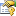# RungeKuttaFehlberg78Integrator Properties

The RungeKuttaFehlberg78Integrator type exposes the following members.Properties
NameDescriptionAbsoluteTolerance
Gets or sets the tolerance which provides a baseline for the error control. By default, this value is Epsilon10.AdaptiveWeights
Gets or sets the weights to be applied to the state elements when computing the error which determines how to adapt the step size. This is in addition to any weights used in the integration algorithm itself. If these weights have not been initialized before Initialize(Double, Double) is called, they will be set to a default value of one.CurrentStepSize
Gets the current (positive) size of the step which the integrator will use for the next integration step.Dimension
Gets the number of dependent variables based on the Dimension of the SystemOfEquations.
(Inherited from NumericalIntegrator.)Direction
Gets or sets whether to integrate forward, increasing the independent variable, or backward, decreasing the independent variable.
(Inherited from NumericalIntegrator.)FinalDependentVariableValues
Gets or sets the values of the dependent variables resulting from the last integration step.
(Inherited from NumericalIntegrator.)FinalIndependentVariableValue
Gets or sets the value of the independent variable after an integration step.
(Inherited from NumericalIntegrator.)InitialDependentVariableValues
Gets or sets the values of the dependent variables prior to an integration step. These values will be updated to the previous FinalDependentVariableValues at the beginning of each integration step.
(Inherited from NumericalIntegrator.)InitialIndependentVariableValue
Gets or sets the value of the independent variable prior to an integration step.
(Inherited from NumericalIntegrator.)InitialStepSize
Gets or sets the initial (positive) size of the step to add to the InitialIndependentVariableValue when performing an integration. By default, the initial value is one.
(Inherited from NumericalIntegrator.)IsThreadSafe
Gets a value indicating whether the methods on this instance are safe to call from multiple threads simultaneously.
(Inherited from NumericalIntegrator.)Iterations
Gets or sets the number of iteration required to adapt the CurrentStepSize to within error tolerance.MaximumIterations
Gets or sets the maximum number of Iterations to allow before forcing the integrator to accept the CurrentStepSize and proceed to the next step.MaximumStepSize
Gets or sets the maximum size of the CurrentStepSize.MinimumStepSize
Gets or sets the (positive) minimum size of the CurrentStepSize.PreviousStepSize
Gets the size of the last step taken by the integrator. This returns the difference between the FinalIndependentVariableValue and the InitialIndependentVariableValue.
(Inherited from NumericalIntegrator.)RelativeTolerance
Gets or sets the tolerance which provides a relative weight for the numerical error based on the dependent variables. By default, this value is Epsilon13.StepDeflationExponent
Gets or sets the exponent to apply to the error when calculating the amount to decrease the size of the step.StepDeflationFactor
Gets or sets a scalar multiplier to apply to the step when decreasing it.StepInflationExponent
Gets or sets the exponent to apply to the error when calculating the amount to increase the size of the step.StepInflationFactor
Gets or sets a scalar multiplier to apply to the step when increasing it.StepSizeBehavior
Gets or sets whether to avoid adapting the step size and instead fix the value of CurrentStepSize.StepSizeInformation
Gets or sets a value indicating whether the CurrentStepSize was changed from the InitialStepSize and, if so, the nature of how it was changed.
(Inherited from NumericalIntegrator.)StepTruncationOrder
Gets or sets the integer exponent specifying the order of magnitude at which to truncate the significant digits in the CurrentStepSize. For instance, to truncate the value to three decimal places, specify a truncation order of -3. To truncate the value to an order of 100, specify a truncation order of 2. To specify the step size should be an integer value, specify a truncation order of 0.SystemOfEquationsTableauSee Also